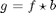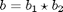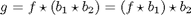Dilation algorithms—structuring element decomposition

This is the second post in my series on algorithm concepts behind the implementation of dilation and erosion in the Image Processing Toolbox. Today I want to talk about structuring element decomposition.

Some useful structuring elements can be represented as the dilation of two or more smaller structuring elements. Dilation implementations can exploit this decomposition of structuring elements into smaller structuring elements by taking advantage of the associativity property of dilation.

That is, suppose image g is the dilation of image f with structuring element b:where the star operator means dilation. And further suppose that b is the dilation of two other structuring elements:Then the original image dilation equation can be rewritten as:In English, the equation above says we can dilate f with structuring element b by:

1. Dilating f first with structuring element b1.

2. Dilating the result of step 1 with structuring element b2.

Let's see how this might help make dilation faster. One of the most commonly-used structuring element shapes is the square. For example, here's a simple 5-by-5 array of 1s that can define a structuring element domain (or neighborhood):

b = ones(5, 5)
b =

1     1     1     1     1
1     1     1     1     1
1     1     1     1     1
1     1     1     1     1
1     1     1     1     1

It turns out that a square (or rectangular) structuring element can be decomposed into two structuring elements: a horizontal line, and a vertical line.

b1 = ones(1, 5)
b1 =

1     1     1     1     1

b2 = ones(5, 1)
b2 =

1
1
1
1
1

You can check that the dilation of b1 and b2 equals b by using the 'full' option of imdilate:

imdilate(b1, b2, 'full')
ans =

1     1     1     1     1
1     1     1     1     1
1     1     1     1     1
1     1     1     1     1
1     1     1     1     1

How does this help us? Well, as I discussed last time, computing dilation directly from its defining equation requires Q comparisons per pixel, where Q is the number of elements in the structuring element domain. The 5-by-5 square structuring element has 25 elements in its domain. But structuring elements b1 and b2 have only five elements each. So you could dilate by one and then again by the other and require only 40% of the comparisons.

Let's look at how structuring element decompositions are computed and represented in the Image Processing Toolbox. The function strel makes a structuring element object. You give strel the name of a shape, such as 'rectangle', plus any parameters required for the shape. When you use strel to make a rectangular structuring element, the resulting object displays information about itself, including the decomposition:

se = strel('rectangle', [5 5])

se =

Flat STREL object containing 25 neighbors.
Decomposition: 2 STREL objects containing a total of 10 neighbors

Neighborhood:
1     1     1     1     1
1     1     1     1     1
1     1     1     1     1
1     1     1     1     1
1     1     1     1     1

You can call the function getsequence (actually a method of the strel class) to get the structuring elements in the decomposition:

decomp = getsequence(se)

decomp =

2x1 array of STREL objects

You use ordinary MATLAB array indexing to get each of the structuring elements from the decomposition:

decomp(1)

ans =

Flat STREL object containing 5 neighbors.

Neighborhood:
1
1
1
1
1

decomp(2)

ans =

Flat STREL object containing 5 neighbors.

Neighborhood:
1     1     1     1     1

Ordinarily, of course, strel and imdilate together handle all of these details for you when you perform image dilation.

Next time I plan to look at some of the other shapes offered by strel and see how they get decomposed.

Published with MATLAB® 7.6

|